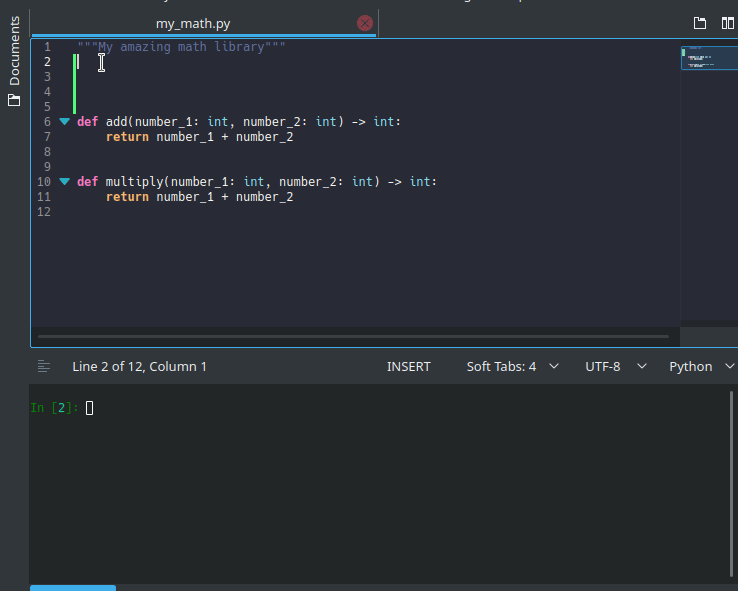# HomeeXamples (AKA: xamples for SEO) is a Python3 library enabling interactable, self-documenting, and self-verifying examples. These examples are attached directly to Python functions using decorators or via separate `MODULE_examples.py` source files.Key Features:

• Simple and Obvious API: Add `@examples.example(*args, **kwargs)` decorators for each example you want to add to a function.
• Auto Documenting: Examples, by default, get added to your functions docstring viewable both in interactive interpreters and when using portray or pdocs.
• Signature Validating: All examples can easily be checked to ensure they match the function signature (and type annotations!) with a single call (`examples.verify_all_signatures()`).
• Act as Tests: Examples act as additional test cases, that can easily be verified using a single test case in your favorite test runner: (`examples.test_all_examples()`).
• Async Compatibility: Examples can be attached and tested as easily against async functions as non-async ones.

What's Missing:

• Class Support: Currently examples can only be attached to individual functions. Class and method support is planned for a future release.

## Quick Start

The following guides should get you up and running using eXamples in no time.

1. Installation - TL;DR: Run `pip3 install examples` within your projects virtual environment.
2. Adding Examples - TL;DR: Add example decorators that represent each of your examples:

```# my_module_with_examples.py
from examples import example

@example(1, number_2=1, _example_returns=2)
def add(number_1: int, number_2: int) -> int:
return number_1 + number_2
```
3. Verify and Test Examples - TL;DR: run `examples.verify_and_test_examples` within your projects test cases.

```# test_my_module_with_examples.py
from examples import verify_and_test_examples

import my_module_with_examples

def test_examples_verifying_signature():
verify_and_test_examples(my_module_with_examples)
```
4. Introspect Examples -

```import examples

from my_module_with_examples import add

I hope you too find `eXamples` useful!